# Binomial Expansion Calculator

Enter values for X, Y, and n for expansion of binomial term using binomial expansion calculator.

Equation: (X + Y) n

Give Us Feedback

## Binomial Expansion Calculator

Binomial expansion calculator expands the binomial expression using the binomial theorem.

## Binomial theorem - Definition/Formula

For any positive integer n, the nth power of the sum of two numbers a and b may be expressed as the sum of n + 1 terms of the form.

The equation of the binomial theorem is,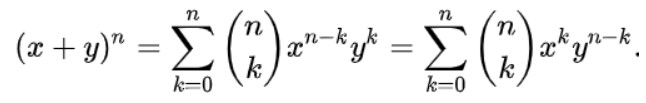Where,

• n ≥ 0 is an integer,
• (n, k) is binomial coefficient.

The fully expanded form of higher exponents can also be calculated using the binomial expansion formula.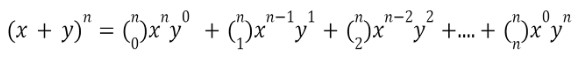## Examples of Binomial theorem:

Example:

What is the expanded form of binomial expression (3 + 5)^4?

Solution:

Step 1:  Expand the expression: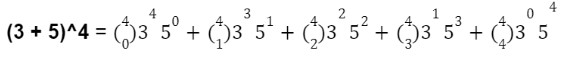Step 2: Find the values of binomial coefficients: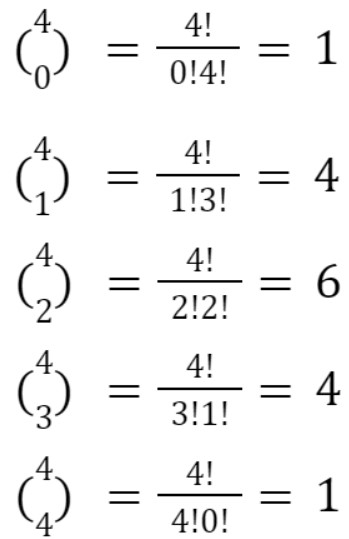Step 3:  put the values of coefficients and solve: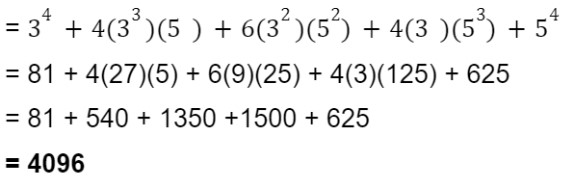The binomial theorem calculator above will give the solution with steps.

### Math Tools